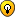## A tricky puzzle

For fans of all other kinds of logic puzzles

### Re: A tricky puzzle

SteveG48 wrote:I agree that the mistress should get the extra.

If you are referring to the specific puzzles/cases in which a man dies and leaves behind a wife, a daughter (case a, or daughters case b) and his parents; and according to the Quran stipulates what is the share of whom as follows:

a)
Wife is 1/8 = 3/24
Daughter 1/2 = 12/24
Father 1/6 = 4/24
Mother 1/6 = 4/24
Total = 23/24

b)
Wife is 1/8 = 3/24
Daughters 2/3 = 16/24 (divide 2/3 share among daughters equally.)
Father 1/6 = 4/24
Mother 1/6 = 4/24
Total = 27/24
rjamil

Posts: 604
Joined: 15 October 2014
Location: Karachi, Pakistan

### Re: A tricky puzzle

rjamil wrote:
SteveG48 wrote:Wife is 1/8 = 3/24
Daughters 2/3 = 16/24 (divide 2/3 share among daughters equally.)
Father 1/6 = 4/24
Mother 1/6 = 4/24
Total = 27/24

27/24? Seriously?
SteveSteveG48
2019 Supporter

Posts: 3082
Joined: 08 November 2013
Location: Orlando, Florida

### Re: A tricky puzzle

SteveG48 wrote:27/24? Seriously?

I think the Goldsmith will have a problem with this oneTarektarek

Posts: 3748
Joined: 05 January 2006

### Re: A tricky puzzle

Hi again,

Actually, these types of equations need to be normalization (either statistically or quantitatively) first in order to achieve precise shares

Hidden Text: Show
Code: Select all
`a) … with only one daughter case:1   1   1   1   23- + - + - + - = --8   2   6   6   24Multiply both the sides by 24 and divide by 23:1   24   1   24   1   24   1   24   23   24- x -- + - x -- + - x -- + - x -- = -- x --8   23   2   23   6   23   6   23   24   23 3   12    4    4-- + -- + -- + -- = 123   23   23   23b) … with two or more daughters (divide two-third of the heirship share in to each daughter equally):1   2   1   1   27- + - + - + - = --8   3   6   6   24Multiply both the sides by 24 and divide by 27:1   24   2   24   1   24   1   24   27   24- x -- + - x -- + - x -- + - x -- = -- x --8   27   3   27   6   27   6   27   24   27 3   16    4    4-- + -- + -- + -- = 127   27   27   27`
Now the above equations show normalized shares. Multiply whatever the actual value of heirship in to both the sides of normalized equation, one will get each share precisely.

R. Jamil
----------
The truth is that English is just a language, simply a medium of communication and interaction. It is not a measure of intelligence or character.
rjamil

Posts: 604
Joined: 15 October 2014
Location: Karachi, Pakistan

### Re: A tricky puzzle

Is that then the solution to the tricky puzzle "Normaization"?(1/2 + 1/3 + 1/9) * 18/17 = 17/18 * 18/17

9/17 + 6/17 + 2/17 = 17/17

tarektarek

Posts: 3748
Joined: 05 January 2006

### Re: A tricky puzzle

tarek wrote:Is that then the solution to the tricky puzzle "Normaization"?

The normalization is the method/process to calculate these type of puzzles.

tarek wrote:(1/2 + 1/3 + 1/9) * 18/17 = 17/18 * 18/17

9/17 + 6/17 + 2/17 = 1

So, your above mentioned equation is shown as the op tricky puzzle's normalization form (i.e., right hand side of the equation must be equals to 1). it's now needs to multiply both the sides by the quantity/amount of heritage in order to achieve the results precisely.
rjamil

Posts: 604
Joined: 15 October 2014
Location: Karachi, Pakistan

### Re: A tricky puzzle

Say chaps, it has been 5 days, and you still haven't given any thought to the poor man's funeral or sorted out how its costs should be divided.
David P Bird
2010 Supporter

Posts: 1043
Joined: 16 September 2008
Location: Middle England

Previous